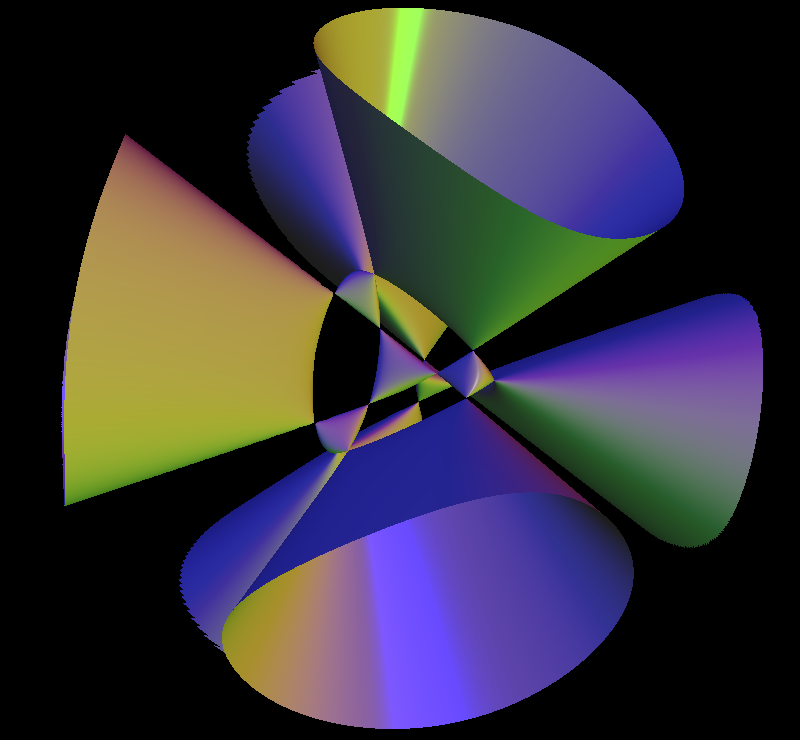# Kummer QuarticReal points of the complex Kummer quartic with its 16 real singularities. (viewed from different angles)

A Kummer surface is any one of a one parameter family of algebraic surfaces defined by the polynomial equation of degree four:

```(x^2 + y^2 + z^2 - aa^2)^2 - lambda*p*q*r*s = 0.

Here aa is any real number.

lambda = (3*aa^2 - 1.0)/(3 - aa^2)
p = 1 - z - sqrt(2)*x
q = 1 - z + sqrt(2)*x
r = 1 + z + sqrt(2)*y
s = 1 + z - sqrt(2)*y
```

The family was described originally by Ernst Eduard Kummer In 1864.

A Kummer surface has sixteen double points, the maximum possible for a surface of degree four in three-dimensional space. For the default case aa = 1.3, all these double points are real and they appear in the visualization as the vertices of five tetrahedra.